## 【C++】模版进阶

article2023/10/2 20:19:47/

### 目录

• 一、非类型模版参数
• 二、模板的特化
• 1、概念
• 2、函数模版特化
• 3、类模板特化
• 1.全特化
• 2.偏特化
• 3.类模板特化应用示例
• 三、模版分离编译
• 1、什么是分离编译
• 2、模板的分离编译
• 3、模板的优缺点

# 一、非类型模版参数

• 类型模版参数：出现在模版参数列表中，跟在class或者typename之类的参数类型名称。
• 非类型模版参数：用一个常量作为类（函数）模版的一个参数，在类（函数）模版中可将该函数当成常量来使用。

``````// 定义一个模板类型的静态数组
template<class T, size_t N = 10>
class array
{
public:
T& operator[](size_t index)
{
return _array[index];
}
const T& operator[](size_t index)const
{
return _array[index];
}

size_t size()const
{
return _size;
}
bool empty()const
{
return 0 == _size;
}

private:
T _array[N];
size_t _size;
};
``````

1. 其中N只能是整形常量，浮点数、类对象以及字符串都不允许作为非类型模板参数的。
2. 非类型的模版参数必须在编译期就能确定结果。
3. 类模版参数解决了typedef只能重定义一种类型的问题。

# 二、模板的特化

## 1、概念

``````class Date
{
public:
Date(int year = 1900, int month = 1, int day = 1)
: _year(year)
, _month(month)
, _day(day)
{}

bool operator<(const Date& d)const
{
return (_year < d._year) ||
(_year == d._year && _month < d._month) ||
(_year == d._year && _month == d._month && _day < d._day);
}

bool operator>(const Date& d)const
{
return (_year > d._year) ||
(_year == d._year && _month > d._month) ||
(_year == d._year && _month == d._month && _day > d._day);
}

friend ostream& operator<<(ostream& _cout, const Date& d)
{
_cout << d._year << "-" << d._month << "-" << d._day;
return _cout;
}

private:
int _year;
int _month;
int _day;
};

// 函数模板 -- 参数匹配
template<class T>
bool Less(T left, T right)
{
return left < right;
}
int main()
{
cout << Less(1, 2) << endl; // 可以比较，结果正确

Date d1(2023, 6, 15);
Date d2(2023, 6, 14);
cout << Less(d1, d2) << endl; // 可以比较，结果正确

Date* p1 = &d1;
Date* p2 = &d2;
cout << Less(p1, p2) << endl; // 可以比较，结果错误
return 0;
}
``````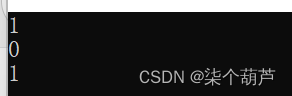## 2、函数模版特化

1. 必须要先有一个基础的函数模版
2. 关键字template后面接一对空的尖括号<>
3. 函数名后跟一对尖括号，尖括号中指定需要特化的类型
4. 函数形参必须要和模版函数的基础参数类型完全相同

``````// 函数模板 -- 参数匹配
template<class T>
bool Less(T left, T right)
{
return left < right;
}

// 对Less函数模板进行特化
template<>
bool Less<Date*>(Date* left, Date* right)
{
return *left < *right;
}
int main()
{
cout << Less(1, 2) << endl; // 可以比较，结果正确

Date d1(2023, 6, 15);
Date d2(2023, 6, 14);
cout << Less(d1, d2) << endl; // 可以比较，结果正确

Date* p1 = &d1;
Date* p2 = &d2;
cout << Less(p1, p2) << endl; // 可以比较，结果错误
return 0;
}
``````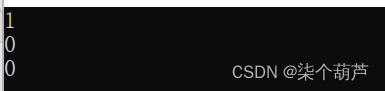``````bool Less(Date* left, Date* right)
{
return *left < *right;
}
``````

## 3、类模板特化

### 1.全特化

``````template<class T1, class T2>
class Data
{
public:
Data()
{
cout << "Data<T1, T2>" << endl;
}
private:
T1 _d1;
T2 _d2;
};
template<>
class Data<int, char>
{
public:
Data()
{
cout << "Data<int, char>" << endl;
}
private:
int _d1;
char _d2;
};
void Test()
{
Data<int, int> d1;
Data<int, char> d2;
}
int main()
{
Test();
return 0;
}
``````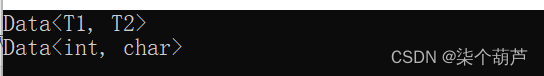### 2.偏特化

``````template<class T1, class T2>
class Data
{
public:
Data()
{
cout << "Data<T1, T2>" << endl;
}
private:
T1 _d1;
T2 _d2;
};
``````

• 部分特化
将模版参数类中的一部分参数进行特化
``````// 将第二个参数特化为int
template <class T1>
class Data<T1, int>
{
public:
Data()
{
cout << "Data<T1, int>" << endl;
}
private:
T1 _d1;
int _d2;
};
``````
• 参数更进一步的限制
偏特化并不仅仅是指特化部分参数，而是针对模板参数更进一步的条件限制所设计出来的一个特化版本。
``````template<class T1, class T2>
class Data
{
public:
Data() {
cout << "Data<T1, T2>" << endl;
}
private:
T1 _d1;
T2 _d2;
};

//将第二个参数特化为int
template <class T1>
class Data<T1, int>
{
public:
Data()
{
cout << "Data<T1, int>" << endl;
}
private:
T1 _d1;
int _d2;
};

//两个参数偏特化为指针类型
template <typename T1, typename T2>
class Data<T1*, T2*>
{
public:
Data() {
cout << "Data<T1*, T2*>" << endl;
}

private:
T1 _d1;
T2 _d2;
};

//两个参数偏特化为引用类型
template <typename T1, typename T2>
class Data <T1&, T2&>
{
public:
Data(const T1& d1, const T2& d2)
: _d1(d1)
, _d2(d2)
{
cout << "Data<T1&, T2&>" << endl;
}

private:
const T1& _d1;
const T2& _d2;
};

void Test2()
{
Data<int, double> d2; // 调用基础的模板
Data<double, int> d1; // 调用特化的int版本
Data<int*, int*> d3; // 调用特化的指针版本
Data<int&, int&> d4(1, 2); // 调用特化的引用版本
}

int main()
{
Test2();
return 0;
}
``````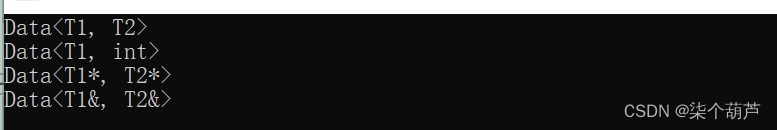### 3.类模板特化应用示例

``````class Date
{
public:
Date(int year = 1900, int month = 1, int day = 1)
: _year(year)
, _month(month)
, _day(day)
{}

bool operator<(const Date& d)const
{
return (_year < d._year) ||
(_year == d._year && _month < d._month) ||
(_year == d._year && _month == d._month && _day < d._day);
}

bool operator>(const Date& d)const
{
return (_year > d._year) ||
(_year == d._year && _month > d._month) ||
(_year == d._year && _month == d._month && _day > d._day);
}

friend ostream& operator<<(ostream& _cout, const Date& d)
{
_cout << d._year << "-" << d._month << "-" << d._day;
return _cout;
}

private:
int _year;
int _month;
int _day;
};
template<class T>
struct Less
{
bool operator()(const T& x, const T& y) const
{
return x < y;
}
};
int main()
{
Date d1(2023, 6, 14);
Date d2(2023, 6, 15);
Date d3(2023, 6, 12);
vector<Date> v1;
v1.push_back(d1);
v1.push_back(d2);
v1.push_back(d3);
// 可以直接排序，结果是日期升序
sort(v1.begin(), v1.end(), Less<Date>());
for (auto e : v1)
{
cout << e << " ";
}
cout << endl;

vector<Date*> v2;
v2.push_back(&d1);
v2.push_back(&d2);
v2.push_back(&d3);
sort(v2.begin(), v2.end(), Less<Date*>());
// 可以直接排序，结果错误，日期还不是升序，而v2中放的地址是升序
// 此处需要在排序过程中，让sort比较v2中存放地址指向的日期对象
// 但是走Less模板，sort在排序时实际比较的是v2中指针的地址，因此无法达到预期
for (auto e : v2)
{
cout << *e << " ";
}
cout << endl;
return 0;
}
````````````//对Less类模板按照指针方式特化
template<>
struct Less<Date*>
{
bool operator()(Date* x, Date* y) const
{
return *x < *y;
}
};
``````# 三、模版分离编译

## 2、模板的分离编译

``````// a.h
template<class T>
T Add(const T& left, const T& right);
void func();

//a.cpp
template<class T>
T Add(const T& left, const T& right)
{
return left + right;
}
void func()
{
cout << "void func()" << endl;
}

//main.cpp
int main()
{
func();
return 0;
}
``````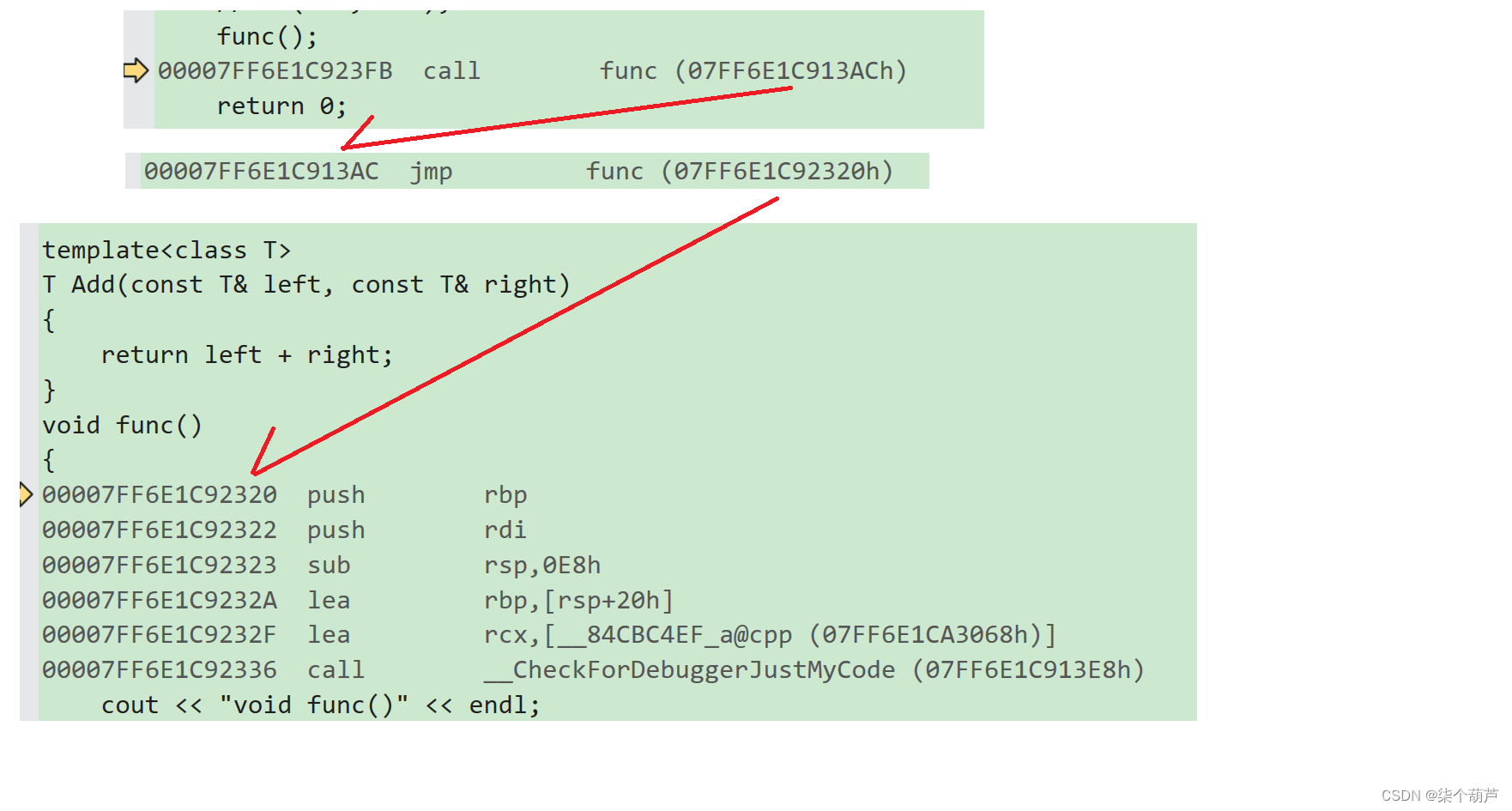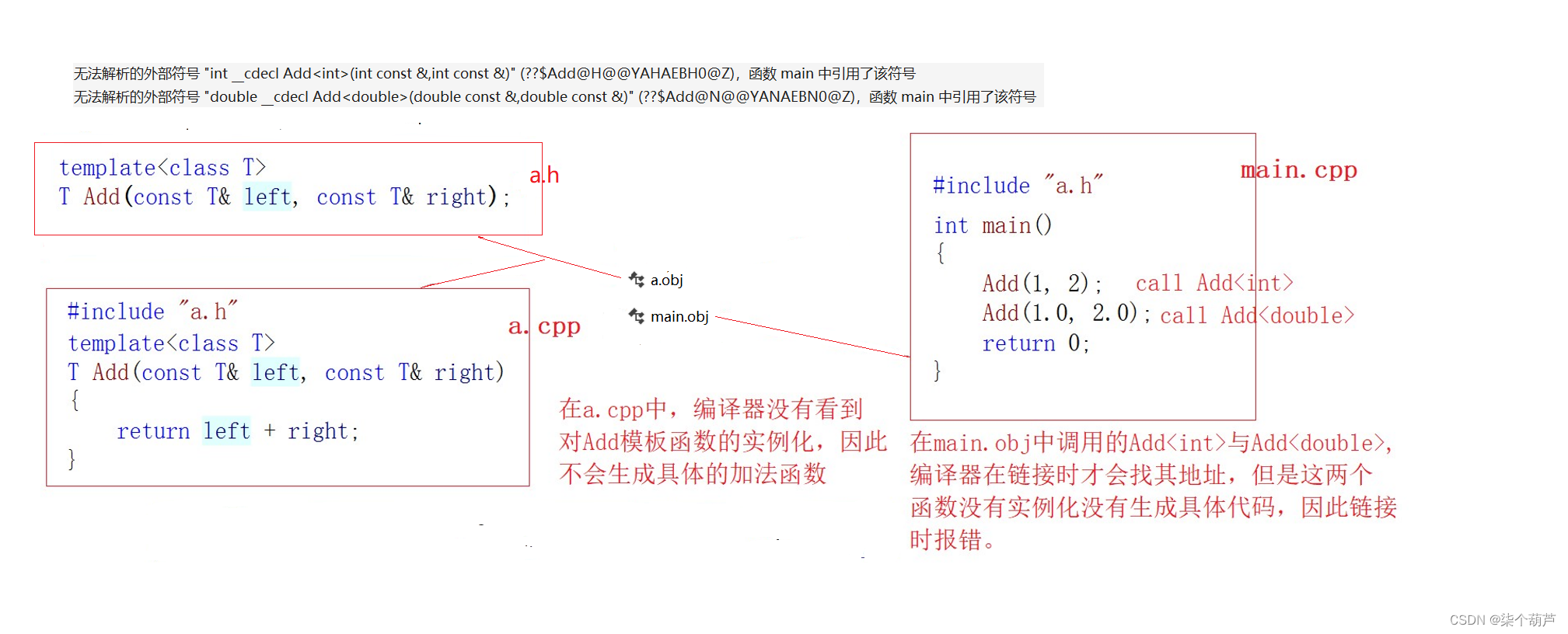1. 将声明和定义放到一个文件 “xxx.cpp” 里面或者xxx.h其实也是可以的。推荐。
2. 模板定义的位置显式实例化。这种方法不实用，不推荐。

## 3、模板的优缺点

1. 模板复用了代码，节省资源，更快的迭代开发，C++的标准模板库(STL)因此而产生。
2. 增强了代码的灵活性。

1. 模板会导致代码膨胀问题，也会导致编译时间变长
2. 出现模板编译错误时，错误信息非常凌乱，不易定位错误

### [进阶]网络通信：UDP通信，一发一收、多发多收

UDP通信 特点&#xff1a;无连接、不可靠通信。不事先建立连接&#xff1b;发送端每次把要发送的数据&#xff08;限制在64KB内&#xff09;、接收端1P、等信息封装成一个数据包&#xff0c;发出去就不管了。Java提供了一个java.net.Datagramsocket类来实现UDP通信。 Datagram…

### Redis简介

Redis是基于内存&#xff0c;也可以基于磁盘持久化nosql数据库&#xff0c;使用c语言开发。 数据存储结构&#xff1a;key-value 安装环境准备 Redis使用c语言开发&#xff0c;需要使用gcc编译程序进行编译。 1&#xff09; 安装gcc a) 从磁盘镜像中进行安装&#xff1a;&…

### 决策树分类算法

#CSDN AI写作助手创作测评 目录 ID3算法 1.算法原理 2.代码实现 3.ID3算法的优缺点分析 C4.5算法 1.原理 2.优缺点 心得感受 决策树表示方法是应用最广泛的逻辑方法之一&#xff0c;它从一组无次序、无规则的事例中推理出决策树表示形式的分类规则。在决策树的内部…

### StarRocks 文章收集

StarRocks在58的实践 StarRocks在58的实践 - 墨天轮StarRocks在58的实践 --2022-06-08https://www.modb.pro/db/639611 StarRocks之系统架构 StarRocks之系统架构 - 墨天轮https://www.modb.pro/db/610300 StarRocks小规模集群部署最佳实践(1/2) 0016.S StarRocks小规模集…

### 2自由度并联仿生腿的制作

1. 运动功能说明 本文实例将实现2自由度并联仿生腿模组运动起来&#xff0c;模拟实现狗腿行走的动作。 2. 结构说明 2自由度并联仿生腿模组是由两个舵机驱动的&#xff0c;它的所有动作都将在两个舵机的配合运动下实现。 3. 运动原理说明 2自由度并联仿生腿模组运动的点位如下…

### 2023 年6月开发者调查统计结果——最流行的技术（1）

2023 年6月开发者调查统计结果——最流行的技术&#xff08;1&#xff09; 本文目录&#xff1a; 一、编程、脚本和标记语言 二、数据库 三、云平台 四、网络框架和技术 五、其他框架和库 六、其他工具 七、集成开发环境 八、异步工具 九、同步工具 ​十、操作系统 …

### MFC扩展库BCGControlBar Pro v33.5亮点 - Ribbon Bar等全新升级

BCGControlBar库拥有500多个经过全面设计、测试和充分记录的MFC扩展类。 我们的组件可以轻松地集成到您的应用程序中&#xff0c;并为您节省数百个开发和调试时间。 BCGControlBar专业版 v33.5已正式发布了&#xff0c;此版本包含了Ribbon&#xff08;功能区&#xff09;自定义…